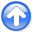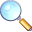Regularization is model stylingNext: SEARCHING THE SEA OF Up: Regularization is model styling Previous: Operator approach to missing

# WELLS NOT MATCHING THE SEISMIC MAP

Accurate knowledge comes from wells, but wells are expensive and far apart. Less accurate knowledge comes from surface seismology, but this knowledge is available densely in space and can indicate significant trends between the wells. For example, a prospective area may contain 15 wells but 600 or more seismic stations. To choose future well locations, it is helpful to match the known well data with the seismic data. Although the seismic data is delightfully dense in space, it often mismatches the wells because there are systematic differences in the nature of the measurements. These discrepancies are sometimes attributed to velocity anisotropy. To work with such measurements, we do not need to track down the physical model, we need only to merge the information somehow to appropriately map the trends between wells and make a proposal for the next drill site. Here, we consider only a scalar value at each location. Taketo be a vector of 15 components, each component being the seismic travel time to some fixed depth in a well. Likewise, letbe a 600-component vector each with the seismic travel time to that fixed depth as estimated wholly from surface seismology. Such empirical corrections are often called fudge factors''. An example is the Chevron oil field in Figure 6.wellseis
Figure 6.
Binning by data push. Left is seismic data. Right is well locations. Values in bins are divided by numbers in bins. (Toldi)The binning of the seismic data in Figure 6 is not really satisfactory when we have available the techniques of missing data estimation to fill the empty bins. Using the ideas of subroutine mis1() , we can extend the seismic data into the empty part of the plane. We use the same principle that we minimize the energy in the filtered map where the map must match the data where it is known. I chose the filterto be the Laplacian operator (actually, its negative) to obtain the result in Figure 7.misseis
Figure 7.
Seismic binned (left) and extended (right) by minimizing energy in.There are basically two ways to handle boundary conditions. First, as we did in Figure 1, by using a transient filter operator that assumes zero outside to the region of interest. Second is to use an internal filter operator. Internal filters introduce the hazard that solutions could be growing at the boundaries. Growing solutions are rarely desirable. In that case, it is better to assign boundary values, which is what I did here in Figure 7. I did not do it because it is better, but did it to minimize the area surrounding the data of interest.

The first job is to fill the gaps in the seismic data. We did such a job in one dimension in Figures 1-5. More computational details later come later. Let us call the extended seismic data.

Think of a map of a model spaceof infinitely many hypothetical wells that must match the real wells, where we have real wells. We must find a map that matches the wells exactly and somehow matches the seismic information elsewhere. Let us define the vector, as shown in Figure 6 sois observed values at wells and zeros elsewhere.

Where the seismic data contains sharp bumps or streaks, we want our final Earth model to have those features. The wells cannot provide the rough features, because the wells are too far apart to provide high-spatial frequencies. The well information generally conflicts with the seismic data at low-spatial frequencies because of systematic discrepancies between the two types of measurements. Thus we must accept thatandmay differ at low-spatial frequencies (where gradient and Laplacian are small).

Our final mapwould be very unconvincing if it simply jumped from a well value at one point to a seismic value at a neighboring point. The map would contain discontinuities around each well. Our philosophy of finding an Earth modelis that our Earth map should contain no obvious footprint'' of the data acquisition (well locations). We adopt the philosophy that the difference between the final map (extended wells), and the seismic informationshould be smooth. Thus, we seek the minimum residual, which is the roughened difference between the seismic dataand the mapof hypothetical omnipresent wells. With roughening operatorwe fit:(12)

along with the constraint that the map should match the wells at the wells. We honor this constraint by initializing the mapto the wells (where we have wells, and zero elsewhere). After we find the gradient direction to suggest some changes to, we simply do not allow those changes at well locations by using a mask. We apply a missing data selector'' to the gradient which zeros out possible changes at well locations. Like with the goal (7), we have:(13)

After minimizingby adjusting, we have our solution.

Now, we prepare some roughening operators. We have already coded a 2-D gradient operator igrad2. Let us combine it with its adjoint to get the 2-D Laplacian operator. (You might notice that the Laplacian operator is self-adjoint,'' meaning that the operator does the same calculation that its adjoint does. Any operator of the formis self-adjoint because. )

system/generic/laplac2.c
  for (i2=0; i2 < n2; i2++) { for (i1=0; i1 < n1; i1++) { j = i1+i2*n1; if (i1 > 0) { if (adj) { p[j-1] -= r[j]; p[j] += r[j]; } else { r[j] += p[j] - p[j-1]; } } if (i1 < n1-1) { if (adj) { p[j+1] -= r[j]; p[j] += r[j]; } else { r[j] += p[j] - p[j+1]; } } if (i2 > 0) { if (adj) { p[j-n1] -= r[j]; p[j] += r[j]; } else { r[j] += p[j] - p[j-n1]; } } if (i2 < n2-1) { if (adj) { p[j+n1] -= r[j]; p[j] += r[j]; } else { r[j] += p[j] - p[j+n1]; } } } } 
Subroutine lapfill() is the same idea as mis1() except that the filterhas been specialized to the Laplacian implemented by module laplac2.

system/generic/lapfill.c
 void lapfill(int niter /* number of iterations */, float* mm /* model [m1*m2] */, bool *known /* mask for known data [m1*m2] */) /*< interpolate >*/ { if (grad) { sf_solver (sf_igrad2_lop, sf_cgstep, n12, 2*n12, mm, zero, niter, "x0", mm, "known", known, "verb", verb, "end"); } else { sf_solver (laplac2_lop, sf_cgstep, n12, n12, mm, zero, niter, "x0", mm, "known", known, "verb", verb, "end"); } sf_cgstep_close (); } 

Subroutine lapfill() can be used for each of our two applications: (1) extending the seismic data to fill space, and (2) fitting the map exactly to the wells and approximately to the seismic data. When extending the seismic data, the initially non-zero componentsare fixed and cannot be changed.

The final map is shown in Figure 8.finalmap
Figure 8.
Final map based on Laplacian roughening.Results can be computed with various filters. I tried bothand. There are disadvantages of each,being too cautious andperhaps being too aggressive. Figure 9 shows the differencebetween the extended seismic data and the extended wells. Notice that forthe difference shows a localized tent pole'' disturbance about each well. For, there could be a large overshoot between wells, especially if two nearby wells have significantly different values. I do not see that problem here.

My overall opinion is that the Laplacian does the better job in this case. I have that opinion because in viewing the extended gradient, I can clearly see where the wells are. The wells are where we have acquired data. We would like our map of the world to not show where we acquired data. Perhaps our estimated map of the world cannot help but show where we have and have not acquired data, but we would like to minimize that aspect.

 A good image of the Earth hides our data acquisition footprint.diffdiff
Figure 9.
Difference between wells (the final map) and the extended seismic data. Left is plotted at the wells (with gray background for zero). Center is based on gradient roughening and shows tent-pole-like residuals at wells. Right is based on Laplacian roughening.To understand the behavior theoretically, recall that in one dimension the filterinterpolates with straight lines andinterpolates with cubics. The reason is that the fitting goal, leads toor; whereas, the fitting goalleads to, which is satisfied by cubics. In two dimensions, minimizing the output ofgives us solutions of Laplace's equation with sources at the known data. It is as ifstretches a rubber sheet over poles at each well; whereas,bends a stiff plate.

Just becausegives smoother maps thandoes not mean those maps are closer to reality. An objectively better choice for the model styling goal is addressed in Chapter. It is the same issue we noticed when comparing Figures 1-5.Regularization is model stylingNext: SEARCHING THE SEA OF Up: Regularization is model styling Previous: Operator approach to missing

2014-12-03